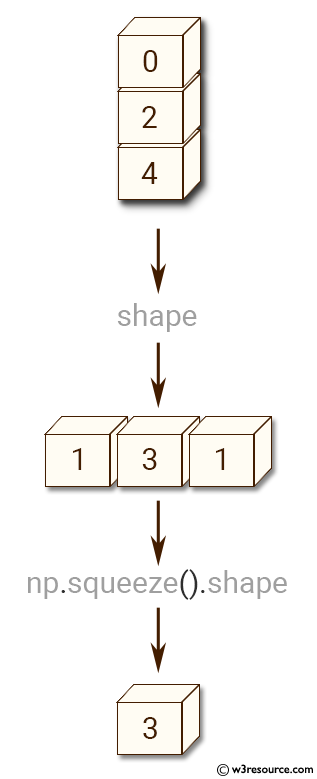# NumPy Array manipulation: squeeze() function

## numpy.squeeze() function

The squeeze() function is used to remove single-dimensional entries from the shape of an array.

Syntax:

`numpy.squeeze(a, axis=None)`Version: 1.15.0

Parameter:

Name Description Required /
Optional
a Input data. Required
axis Selects a subset of the single-dimensional entries in the shape. If an axis is selected with shape entry greater than one, an error is raised. Optional

Return value:

squeezed [ndarray] The input array, but with all or a subset of the dimensions of length 1 removed. This is always a itself or a view into a.

Raises: ValueError - If axis is not None, and an axis being squeezed is not of length 1

Example-1: numpy.squeeze() function

``````>>> import numpy as np
>>> a = np.array([[, , ]])
>>> a.shape
(1, 3, 1)
>>> np.squeeze(a).shape
(3,)
``````

Pictorial Presentation:Example-2: numpy.squeeze() function

``````>>> import numpy as np
>>> a = np.array([[, , ]])
>>> np.squeeze(a, axis=0).shape
(3, 1)
>>> np.squeeze(a, axis=1).shape
Traceback (most recent call last):
File "<stdin>", line 1, in <module>
File "/usr/local/lib/python3.6/dist-packages/numpy/core/fromnumeric.py", line 1201, in squeeze
return squeeze(axis=axis)
ValueError: cannot select an axis to squeeze out which has size not equal to one
>>> np.squeeze(a, axis=2).shape
(1, 3)
``````

Python - NumPy Code Editor:

Previous: expand_dims()
Next: Changing kind of array asarray()

﻿